# PyCG 1: Introduction

Although there are a handful of tools for visualizing data in Python, nothing can come close to OpenGL regarding performance and interaction. As for myself, I have found two cases where I have to use OpenGL inevitably: there was once when I need to write a demonstration of solving partial differential equations to animate liquid surface; and there was another time when I needed to build an interactive program that shows what it is like if a projector is presenting its image on a spherical surface. I will elaborate on these two cases during this series of tutorials.

Because there are numerous wonderful tutorials on OpenGL, such as learnopengl, and that the function calls in Python and C++ are almost identical, I will not put my emphasis on writing about how to program OpenGL code from scratch. Instead, I will focus on how to use OpenGL and Python tool chain to solve real world problems effectively.

Some helpful links are attached below.

## Essential packages

There are two key packages that we are using throughout the entire series.

1. PyOpenGL

PyOpenGL is the most common cross platform Python binding to OpenGL and related APIs. The binding is created using the standard ctypes library, and is provided under an extremely liberal BSD-style Open-Source license. A package called PyOpenGL_accelerate is aimed at accelerating the execution speed of this package.

2. glfw

This module provides Python bindings for GLFW (on GitHub: glfw/glfw). It is a ctypes wrapper which keeps very close to the original GLFW API. (The system needs to be installed with glfw3 before using this package.)

PyOpenGL allows us to access basic OpenGL functionalities, and glfw enables us to create windows and handle events on multiple platforms.

## OpenGL coordinate system

This page on learnopengl described the coordinate system in detail. In short, we should at least know that OpenGL operates on Normalized Device Coordinate (NDC), which specifies that the range of values of $$x$$, $$y$$ and $$z$$ axes should be within $$[-1, 1]$$. Anything out of this range will not be visible.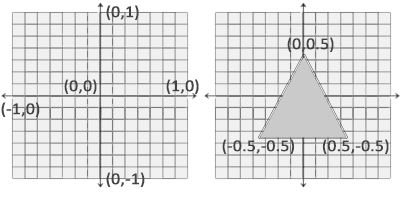NDC (source: learnopengl)

The 3D coordinate system of OpenGL is also a bit different from what can be seen on textbooks, because the positive direction of $$z$$ axis is pointing outward from the screen.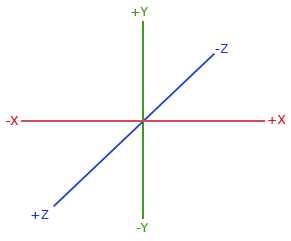OpenGL coordinate system (source: learnopengl)

## The modern OpenGL rendering pipeline

Modern OpenGL almost always works with triangles. Each triangle region is rasterized into pixels called fragments, which will be assigned with color.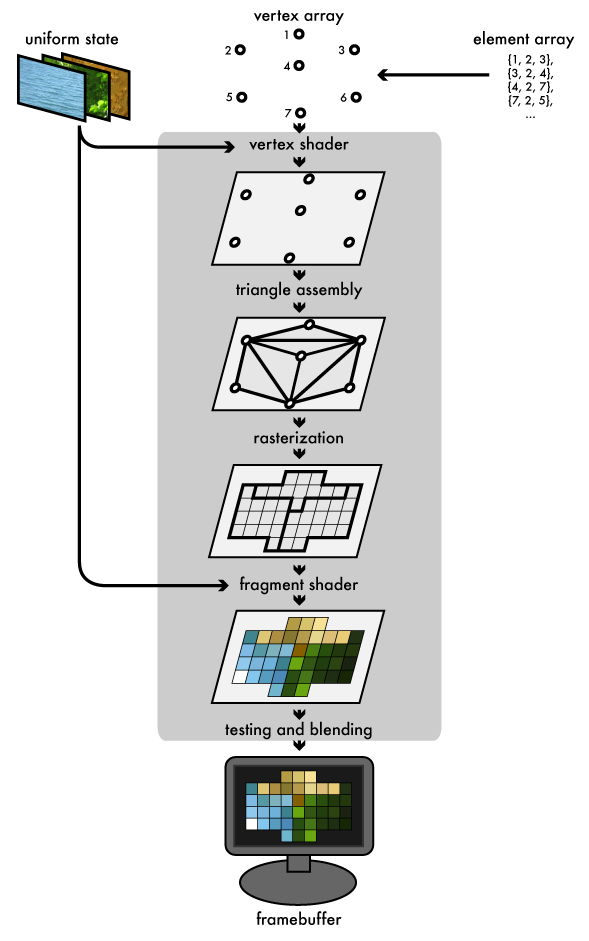OpenGL rendering pipeline (source: Joe Groff)

These procedures are processed by GPU that runs customized programs called shaders. They are written in a C-like language called GLSL. There are three types of shaders in the graphics pipeline, which are listed as follows:

1. Vertex shader: The vertex shader is the programmable shader stage in the rendering pipeline that handles the processing of individual vertices. vertex shaders are fed with vertex attribute data, as specified from a vertex array object by a drawing command.

2. Geometry shader: A geometry shader takes as input a set of vertices that form a single primitive (e.g. a point or a triangle). The geometry shader can then transform these vertices as it sees fit before sending them to the next shader stage. It is able to transform the vertices to completely different primitives possibly generating much more vertices than were initially given.

3. Fragment shader: A fragment shader is the shader stage that will process a Fragment generated by the rasterization into a set of colors and a single depth value. The fragment shader is the OpenGL pipeline stage after a primitive is rasterized. For each sample of the pixels covered by a primitive, a “fragment” is generated.

Geometry shaders are optional, but vertex and fragment shaders are essential for a graphics program to run correctly. This page briefly introduces how to write vertex and fragment shaders.

## Communication between host and device

OpenGL assumes a heterogeneous architecture, where GPUs (devices) cannot access host (CPU) memory directly. Therefore, we need a special approach to pass data from host to device. There are mainly two ways to do so:

1. Vertex buffer objects (VBO) and vertex attribute objects (VAO) : The combination of these two allows one to pass an array from host to device with some additional hints. The array will be partitioned into vertices, where each vertex holds all data needed for rendering itself.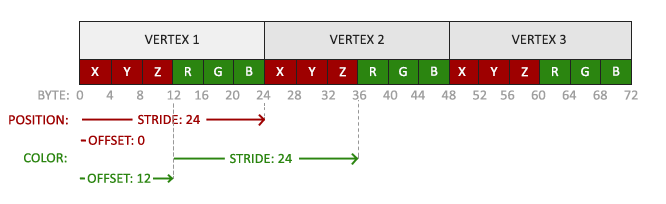VBO and VAO (source: learnopengl)

In this example, an array of 18 single-precision floats are divided into 3 vertices, where each vertex holds two 3-vectors, namely position and color. The meaning of stride and offset in this case are straightforward.

2. Uniform: It allows one to pass a single data element from host to device. For example, it is possible to pass a integer, a vector or a matrix via uniform.

## Hello triangle!

Let’s conclude this introduction with a simple hello triangle program, which generates the following window.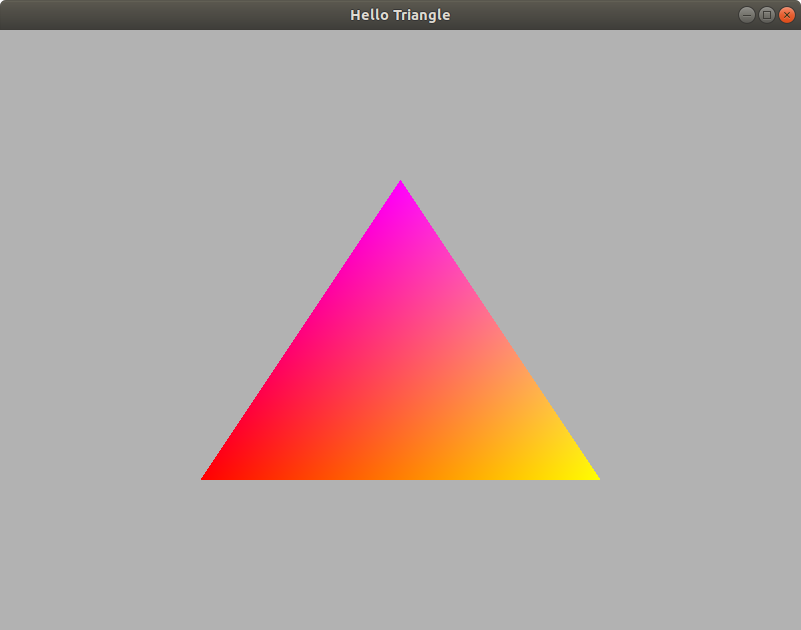Hello triangle

The code is as follows. On Ubuntu, I find it a bit odd because the window is blank until I resize it (because window_resize_callback is called internally with invalid parameters), but it works fine on macOS.

from OpenGL.GL import *
from OpenGL.arrays.vbo import VBO

# because the Python version of glfw changes its naming convention,
# we will always call glfw functinos with the glfw prefix
import glfw
import numpy as np
import platform
import ctypes

windowSize = (800, 600)
windowBackgroundColor = (0.7, 0.7, 0.7, 1.0)

triangleVertices = np.array(
[-0.5, -0.5, 0.0,  # pos 0
1.0, 0.0, 0.0,  # color 0
0.5, -0.5, 0.0,  # pos 1
1.0, 1.0, 0.0,  # color 1
0.0, 0.5, 0.0,  # pos 2
1.0, 0.0, 1.0  # color 2
],
np.float32  # must use 32-bit floating point numbers
)

vertexShaderSource = r'''
#version 330 core
layout (location = 0) in vec3 aPos;
layout (location = 1) in vec3 aColor;
out vec3 bColor;
void main()
{
gl_Position = vec4(aPos, 1.0);
bColor = aColor;
}
'''

fragmentShaderSource = r'''
#version 330 core
in vec3 bColor;
out vec4 FragColor;
void main()
{
FragColor = vec4(bColor, 1.0f);
}
'''

# compile a shader
# returns the shader id if compilation is successful
# otherwise, raise a runtime error
def compile_shader(shaderType, shaderSource):
shaderId = glCreateShader(shaderType)
glShaderSource(shaderId, shaderSource)
glCompileShader(shaderId)
success = glGetShaderiv(shaderId, GL_COMPILE_STATUS)
if not success:
infoLog = glGetShaderInfoLog(shaderId)
print('shader compilation error\n')
print('shader source: \n', shaderSource, '\n')
print('info log: \n', infoLog)
raise RuntimeError('unable to compile shader')
return shaderId

def debug_message_callback(source, msg_type, msg_id, severity, length, raw, user):
msg = raw[0:length]
print('debug', source, msg_type, msg_id, severity, msg)

def window_resize_callback(theWindow, width, height):
global windowSize
windowSize = (width, height)
glViewport(0, 0, width, height)

if __name__ == '__main__':

# initialize glfw
glfw.init()

# set glfw config
glfw.window_hint(glfw.CONTEXT_VERSION_MINOR, 3)
glfw.window_hint(glfw.CONTEXT_VERSION_MAJOR, 3)
glfw.window_hint(glfw.OPENGL_PROFILE, glfw.OPENGL_CORE_PROFILE)

if platform.system().lower() == 'darwin':
# not sure if this is necessary, but is suggested by learnopengl
glfw.window_hint(glfw.OPENGL_FORWARD_COMPAT, GL_TRUE)

# create window
theWindow = glfw.create_window(windowSize, windowSize, 'Hello Triangle', None, None)
# make window the current context
glfw.make_context_current(theWindow)

if platform.system().lower() != 'darwin':
# enable debug output
# doesn't seem to work on macOS
glEnable(GL_DEBUG_OUTPUT)
glDebugMessageCallback(GLDEBUGPROC(debug_message_callback), None)
# set resizing callback function
glfw.set_framebuffer_size_callback(theWindow, window_resize_callback)

# create VBO to store vertices
verticesVBO = VBO(triangleVertices, usage='GL_STATIC_DRAW')
verticesVBO.create_buffers()

# create VAO to describe array information
triangleVAO = glGenVertexArrays(1)

# bind VAO
glBindVertexArray(triangleVAO)

# bind VBO
verticesVBO.bind()
# buffer data into OpenGL
verticesVBO.copy_data()

# configure the fist 3-vector (pos)
# arguments: index, size, type, normalized, stride, pointer
# the stride is 6 * 4 because there are six floats per vertex, and the size of
# each float is 4 bytes
glVertexAttribPointer(0, 3, GL_FLOAT, GL_FALSE, 6 * 4, ctypes.c_void_p(0))
glEnableVertexAttribArray(0)

# configure the second 3-vector (color)
# the offset is 3 * 4 = 12 bytes
glVertexAttribPointer(1, 3, GL_FLOAT, GL_FALSE, 6 * 4, ctypes.c_void_p(3 * 4))
glEnableVertexAttribArray(1)

# unbind VBO
verticesVBO.unbind()
# unbind VAO
glBindVertexArray(0)

# compile shaders
vertexShaderId = compile_shader(GL_VERTEX_SHADER, vertexShaderSource)
fragmentShaderId = compile_shader(GL_FRAGMENT_SHADER, fragmentShaderSource)
# link shaders into a program
programId = glCreateProgram()
glAttachShader(programId, vertexShaderId)
glAttachShader(programId, fragmentShaderId)
glLinkProgram(programId)
linkSuccess = glGetProgramiv(programId, GL_LINK_STATUS)
if not linkSuccess:
infoLog = glGetProgramInfoLog(programId)
print('program linkage error\n')
print('info log: \n', infoLog)
raise RuntimeError('unable to link program')

# delete shaders for they are not longer useful
glDeleteShader(vertexShaderId)
glDeleteShader(fragmentShaderId)

# keep rendering until the window should be closed
while not glfw.window_should_close(theWindow):
# set background color
glClearColor(*windowBackgroundColor)
glClear(GL_COLOR_BUFFER_BIT)

# use our own rendering program
glUseProgram(programId)

# bind VAO
glBindVertexArray(triangleVAO)
# draw vertices
glDrawArrays(GL_TRIANGLES, 0, triangleVertices.size)
# unbind VAO
glBindVertexArray(0)

# tell glfw to poll and process window events
glfw.poll_events()
# swap frame buffer
glfw.swap_buffers(theWindow)

# clean up VAO
glDeleteVertexArrays(1, [triangleVAO])
# clean up VBO
verticesVBO.delete()

# terminate glfw
glfw.terminate()



The source code can also be found in the Github repository.